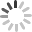Loading...

# How to Create Math Quizzes With Symbols and Mathematical Formulae

ProProfs comes with a sturdy maths formula editor that allows you to create all types of mathematical quizzes. Our editor is based on the standard MathML, so it runs on any browser (Firefox, Explorer, Chrome, etc.) and operating system (Windows, Linux, Mac, etc.).

You can add mathematical symbols and formulas to the question text and also the options and choices.

Follow the steps below to learn how to add mathematical symbols and formulas to the question text of a quiz:

Step 1: Navigate to "Settings" -----> "Advanced" -----> "Math Editor," and turn the toggle to "Yes."Step 2: In the question editor, select the Math editor icon.Step 3: Select and add symbols to create mathematical formulas and then click "Insert Formula" to add it to the question.How to add tables to the question:

Step 1: Click the table icon from the question editor.Step 2:  Select the dimensions and properties of the table and click "Ok."Here's how the table will appear in the editor:Follow the steps below to learn how to add mathematical symbols and formulas to the options and choices of a quiz:

*Before starting, make sure you have turned on the "math editor" from the advanced settings for the quiz.

Step 1: Click the "Media" button above the option editor.Step 2: Select "Math editor" icon.Step 3:  To access the symbol library, click the menu button as shown in the image below.Step 4: To select the appropriate symbol group, click the drop-down menu button and chose a symbol. Add all symbols you want, to create a formula and then click "Insert Formula" to add it to the options field.Step 5: Click "Save," and then preview the question or the quiz to see the changes.Here's a preview of the question:Related Articles:

Introduction to the Quiz Editor
How to Create a Cloze Test
How do I Bulk-Import Questions from Excel?